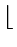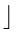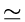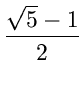Next:3.5.3 Universal HashingUp:3.5 Hashing FunctionsPrevious:3.5.1 Division Method

## 3.5.2 Multiplication Method

There are two steps:
1.
Multiply the key k by a constant A in the range 0 < A < 1 and extract the fractional part of kA
2.
Multiply this fractional part by m and take the floor.
h(k) =m(kA mod 1)where
 kA mod 1 = kA -kAh(k) =m(kA -kA)• Advantage of the method is that the value of m is not critical. We typically choose it to be a power of 2:
m = 2p
for some integer p so that we can then easily implement the function on most computers as follows:

Suppose the word size = w. Assume that k fits into a single word. First multiply k by the w-bit integerA.2w. The result is a 2w - bit value

r12w + r0
where r1 is the high order word of the product and r0 is the low order word of the product. The desired p-bit hash value consists of the p most significant bits of r0.
• Works practically with any value of A, but works better with some values than the others. The optimal choice depends on the characteristics of the data being hashed. Knuth recommends
• A= 0.6180339887...  (Golden Ratio)Next:3.5.3 Universal HashingUp:3.5 Hashing FunctionsPrevious:3.5.1 Division Method
eEL,CSA_Dept,IISc,Bangalore